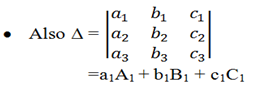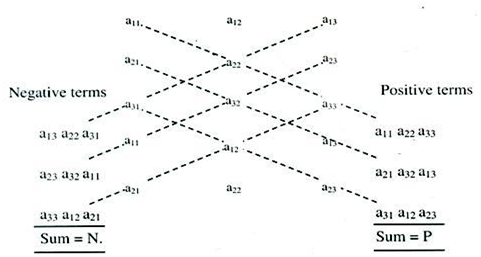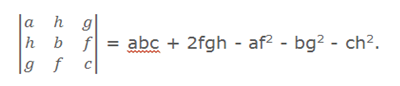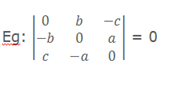Click to Chat

1800-1023-196

+91-120-4616500

CART 0

• 0

MY CART (5)

Use Coupon: CART20 and get 20% off on all online Study Material

ITEM
DETAILS
MRP
DISCOUNT
FINAL PRICE
Total Price: Rs.

There are no items in this cart.
Continue Shopping• Complete JEE Main/Advanced Course and Test Series
• OFFERED PRICE: Rs. 15,900
• View Details

```Revision Notes on Determinants and System of Equations

Minor of the element at the ith row is the determinant obtainedby deleting the ith row and the jthcolumn

The cofactor of this element is (-1)i+j (minor).where A1, B1 and C1 are the cofactors of a1, b1 and c1 respectively.

The determinant can be expanded along any row or column, i.e. Δ = a2A2 + b2B2 + c2C2 or Δ = a1A1 + a2A2 + a3A3 etc.

The following result holds true for determinants of any order: aiAj + biBj + ciCj  = Δ  if i = j,  = 0   if i ≠ j.

Some important properties of matrices and determinants:

1) If rows be changed into columns and columns into the rows, then the values of the determinant remains unaltered.

2) If any two row (or columns) of a determinant are interchanged, the resulting determinant is the negative of the original determinant.

3) If two rows (or two columns) in a determinant have corresponding elements that are equal, the value of determinant is equal to zero.

4) If each element in a row (or column) of a determinant is written as the sum of two or more terms then the determinant can be written as the sum of two or more determinants.

5) If to each element of a line (row or column) of a determinant, some multiples of corresponding elements of one or more parallel lines are added, then the determinant remains unaltered.

6) If each element in any row (or any column) of determinant is zero, then the value of determinant is equal to zero.

7) If a determinant D vanishes for x = a, then (x - a) is a factor of D, in other words, if two rows (or  two columns) become identical for x = a, then (x-a) is a factor of D.

8) In general, if r rows (or r columns) become identical when a is substituted for x, then (x-)r-1 is a factor of D.

The minor of an element of a determinant is again a determinant (of lesser order) formed by excluding the row and column of the element.

The determinant can be evaluated by multiplying the elements of a single row or a column with their respective co-factors and then adding them, i.e.

Δ = ∑mi=1  aij .Cij,  j = 1, 2,      ...... m.

= ∑mj=1  aij.Cij,   i = 1, 2,         ...... m.

Sarrus Rule: This is the rule for evaluating the determinant of order 3. The process is as given below:

1) Write down the three rows of a determinant.

2) Rewrite the first two rows.

3) The three diagonals sloping down the right give the three positive terms and the three diagonals sloping down to the left give the three negative terms.Multiplication of two Determinants:

1) Two determinants can be multiplied together only if they are of same order.

2) Take the first row of determinant and multiply it successively with 1st, 2nd& 3rd rows of other determinant.

3) The three expressions thus obtained will be elements of 1st row of resultant determinant. In a similar manner the element of 2nd& 3rd rows of determinant are obtained.

Symmetric determinant: The elements situated at equal distance from the diagonal are equal both in magnitude and sign.Eg:In a skew symmetric determinant, all the diagonal elements are zero and   the elements situated at equal distance from the diagonal are equal in   magnitude but opposite in sign. The value of a skew symmetric   determinant of odd order is zero.If the elements of a determinant are in cyclic arrangement, such a determinant is termed as a Circulant determinant.

A system of equations AX = D is called a homogeneous system if D = O. Otherwise it is called a non-homogeneous systems of equations.

If the system of equations has one or more solutions, then it is said to be a consistent system of equations, otherwise it is an inconsistent system of equations.

If A is a non-singular matrix, then the system of equations given by

AX = D has a unique solutions given by X = A-1 D.

If A is a singular matrix, and (adjA) D = O, then the system of equations given by AX = D is consistent, with infinitely many solutions.

If A is a singular matrix, and (adjA) D ≠ O, then the system of equation given by AX = D is inconsistent.

Let AX = O be a homogeneous system of n linear equation with n unknowns. Now if A is non-singular then the system of equations will have a unique solution i.e. trivial solution and if A is singular then the system of equations will have infinitely many solutions.

```### Course Features

• 728 Video Lectures
• Revision Notes
• Previous Year Papers
• Mind Map
• Study Planner
• NCERT Solutions
• Discussion Forum
• Test paper with Video Solution